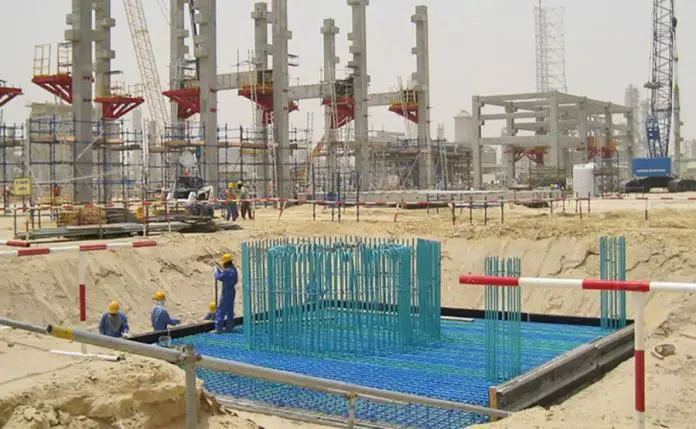# How to Calculate the Settlement of Spread Foundation (EC7 Part 2)In this article, we will discuss an approach described in Annex E of EN 1997-2:2006 (Eurocode 7 Part 2) for determining the elastic settlement of spread foundations. This is based on a semi-empirical method and the result of the MPM test (Menard Pressuremeter Test).

The formula for evaluating the settlement of a spread footing is given below;

where;
Bo is a reference width of 0.6 m;
B is the width of the foundation;
λd , λc are shape factors given in Table E.2;
α is a rheological factor given in Table E.3;
Ec is the weighted value of EM immediately below the foundation;
Ed is the harmonic mean of EM in all layers up to 8 × B below the foundation;
σv0 is the total (initial) vertical stress at the level of the foundation base;
q is the design normal pressure applied on the foundation.

The Table E.2 and E.3 of EC7-2 are given below;

## Solved Example

A 2m x 2m square footing is carrying a quasi-permanent SLS combination load of 1040 kN. The Menard Pressuremeter moduli values are as given in the sketch below. Calculate the settlement of the foundation, if the unit weight of the first layer of soil is 18.0 kN/m3.

Solution

σv0 = 18 kN/m3 × 1.2m = 21.6  kN/m2
q = 1040 kN / (2m × 2m) = 260  kN/m2
B = 2.0m (width of the foundation)
λd = 1.12 (square foundation)
λc = 1.1 (square foundation)
α = 0.67 (normally consolidated clay)
Ec = 16.8 MPa
Ed = 3/[(1/16.8) + (1/27) + (1/33)] =  23.447 MPa
Substituting these values into the settlement equation;

Therefore, the settlement of the foundation is 5.358mm.